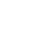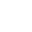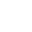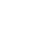﻿ DEPARTMENT OF AUDIO & VISUAL ARTS

# Mathematics and Art

Teaching Staff: Panagopoulos Michail
Course Category: General Background
Course Type: Compulsory
Course Language: Greek
Delivery method: Lectures
Semester: 1st
ECTS: 6
Teaching Units: 4
Teaching Hours: 4
Teaching Structure:
Lectures26
Tutoring Lectures26
Literature Study and Analysis64
Practice and Preparation34
Course Total (ECTS: 6)150

Prerequisite to / Recommended to: TEC414, (AVA745), THE806

Short Description:

This course analyses the relation between mathematics and art through the presentation of specific examples, as well as the basic theory, proving their bonds and interdependence. The course is organised in three basic units (painting, formative arts, and music), in each of which the basic concepts are presented, together with examples of celebrated works, in conjunction with the analysis of the respective mathematical concepts. In the context of the course, special emphasis is placed on the application of the aforementioned concepts through examples and drills. In parallel, special tutorials are provided concerning reviewing basic mathematics that are needed in audiovisual technology.

Objectives - Learning Outcomes:

Scope of the course is to provide to the students an overview of the traditional relation between mathematics and art. To understand the connection between the consistency of science and aesthetics and harmony.

After the attendance of the course the student will be able to:

• spot and identify the golden ratio and symmetries to artefacts and artworks
• understand fractals and their connection to artworks
• understand ratios to music harmony
• to draw functions and curves
• understand basic algebra for computer programming

Syllabus:

1st Week

Course introduction. General description of the syllabus, course evaluation and the compulsory project. Introduction to Golden Ratio: definition – properties of the number Φ.

TUTORIAL: Basic mathematics overview (functions, coordinate system). Basic function graphics (strait lines, parabola, exponential etc.).

2nd Week

Doubling the cube – Fibonacci sequence – Pascal triangle and their relation to Golden Ratio. Golden ratio construction on a line segment and construction of a golden rectangle. Golden triangles, Fibonacci spirals, golden ratio examples – “The Vitruvian Man”.

TUTORIAL: Golden ratio calculation, proof of Φ properties, proof of geometric construction of golden ratio.

3rd Week

Symmetry: Definition – reflection symmetry, rotation symmetry, translation symmetry. Team theory (equivalence of symmetries).

TUTORIAL: Triagular functions, triangular cyrcle. Sinus functions, wave function.

4th Week

Symmetry (cont). Shape symmetry (triangle, rectangle). Rosete symmetry.

TUTORIAL: Logarithms, logarithmic function. Cirle equation.

5th Week

Symmetry of solids (tetrahedron, cube). Frieze symmetry (7 types of symmetry). Wallpaper symmetry (17 symmetries).

TUTORIAL: Symmetry exercises

6th Week

Fractals – Chaotic systems – selfsimularity. Golden triangle, Weierstrass function, Durer pentagon, Von Koch snowflake.

TUTORIAL: Conics – ellipse, parabola, hyperbola. Spirals (logarithmic, linear)

7th Week

Fractals (cont). Sierpinski triangle, Sierpinski carpet. Julia sets, Mandelbrot sets. Fractal dimension. Fractal applications

TUTORIAL: Von Koch snowflake perimeter and area calculation

8th Week

Escher: his work. Symmetry in Escher paintings – Escher tilings

TUTORIAL: Fractal dimension calculation of basic shapes

9th Week

Escher (cont): selfsimularity in Escher works – impossible shapes

TUTORIAL: Introduction to matrices. Definitions, properties – operations with matrices, matrix multiplication

10th Week

Introduction to Music and Math: Pythagoras and fractions, monohord, tone, semitone.

TUTORIAL: Transpose matrices, invert matrix, determinants, systems and determinants.

11th Week

Music and Math (cont): Fourier analysis – twelve-tone method (Schoenberg) - Xenakis

TUTORIAL: Examples of application of mathematical curves to art.

12th Week

General overview of the relation between art and mathematics – mathematics as inspiration to art. Examples (origami, mandala, rosetes, kaleidoscope etc.). Topology and its applications to architechture and scalpture.

TUTORIAL: Analysis Fourier exercises

13th Week

General overview of the course – previous exams demonstration

Suggested Bibliography:

Paul A. Calter. (2006). Squaring the Circle

Sasho Kalajdzievski (2008). Math and Art – An introduction to Visual Mathematics

Felipe Cucker (2013). Manifold Mirrors – The Crossing Paths of the Arts and Mathematics

Teaching Methods:

Teaching is taking place in the classroom where the basic theory is presentment together with provided examples. The tutorial part of the course includes proofs, drills and analysis of theory details.

Evaluation Methods:

The evaluation is a result of the final written exam and a compulsory project.

 << < March 2023 > >> Mo Tu We Th Fr Sa Su 1 2 3 4 5 6 7 8 9 10 11 12 13 14 15 16 17 18 19 20 21 22 23 24 25 26 27 28 29 30 31
Today, Saturday 25-03-2023Text To SpeechText ReadabilityColor Contrast